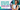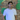Pass Any Exam Online Now & Pay After Passing Exam. Contact Now
GED Math Questions

# What Kind of Math is on the GED? Guide 2022

Oct 16, 2022 • 4 mins readMany students face difficulty in math, one of the four subjects on the GED test, and it is like learning a new foreign language. However, it is not challenging as it looks; with great question practice and resources, anyone can be mastered this section.

Studying for the GED test is way easier because you know which topics you need to cover for the exam, and you can break them into understandable subparts. You can prepare each section separately.

The GED math test will challenge your basic knowledge of mathematics, geometry, algebra, graphs, and functions. Once you master each part with practice, you will be confident enough to pass the GED math test without minimal effort.

## General Tips: GED Math Section

The following tip will be helpful for candidates who are planning to take the GED test.

### Tip 1:

As a GED aspirant, you do not need to memorize the math formulas before the test. You just need to learn the basic concepts behind formulas and their applications. You are expected to practice the basic concepts questions, and you will be good to take the math test.

A descriptive formula reference sheet will be provided in the exam in which all the relevant formulas will be mentioned.

### Tip 2:

You must review all the related math sections and practice general math questions. Plus, you have to focus on standard measurement units and real-life applications.

### Tip 3:

The exam is conducted in 2 parts, and in the second section, you are allowed to use the scientific calculator. The math test includes multiple-choice questions, drag and drop, drop-down, fill-in-the-blanks, and others.

The given options in multiple choice questions may guide you to provide the clue of the correct answer. They may be able to mislead you simultaneously. Make yourself confident enough to solve the problems on your own.

If you face any difficulty judging the right answers, you can choose the right answer by using the elimination method.

### Tip 4:

As the math part is conducted in two sections, you will allow using the scientific calculator in the 2nd part only.

It means candidates need to learn basic ideas of math concepts as well as how to use the scientific calculator in the exam. You do not need to buy a separate calculator for the exam; it will be provided during the math test.

## What Are the Significant Types of Math to Study?

The GED math test is divided into 4 major parts as follows.

### 1. Basic Math:

In the section, you are expected to perform the essential mathematics operation, including addition, subtraction, multiplication, and division using a scientific calculator.

You need to prepare yourself to practice with fractions, decimals, percentages, roots, and square roots, and exponents & powers. You may face word problems in the math test where you must know to perform the math operation to solve such programs.

### 2. Geometry:

Geometry involves the concept of shapes and objects. Candidates need to understand the use of suitable geometry formulas related to shapes. Candidates can look at a shape drown in the geometry problem.

You do not have to memorize all the formulas related to geometry as you will be provided with the formulas sheet, which will help you to calculate surface area, volumes, radius, diameter, and others of a particular shape.

### 3. Algebra:

To get through this section, candidates must be able to figure out the required value of variables in an equation, including quadratic and cubic or linear equations. Apart from it, you must know how to write a basic equation with variables on a word problem.

### 4. Graphs and Functions:

Students have to create a great understanding of graphs and functions. They should be able to read and analyze the information provided through graphs and charts.

You should have practice questions related to mean, median, mode, range, probability, and statistics, ways to write data in tables, and how to organize data in tables.

## How to Study to Pass Your GED?

Now, after going through this article, you know the basic concepts in GED Maths exam and the need to focus on each section separately.

The GED official website provides authentic and quality study materials for students planning to take the GED test in the future. The GED flash is a platform where you can access the thousands of sample math practice tests and teach them, which are great for clearing your vision and boosting your confidence.

Preparing and practicing the math test section by section is highly recommended until you have mastered it.

Once you are done with the preparation of all sections, you can attend the GED-ready full-length math practice test and track your preparation. This is the best recipe to enhance your GED test scores and improve your chances of clearing the math test in one shot.Amit Masih
Amit is a freelance content writer. He helps IT agencies, bloggers and digital firms with engaging, conversational and audience-specific content. He has more than 4 years of industry experience.